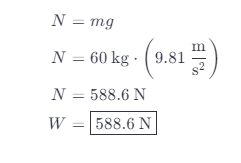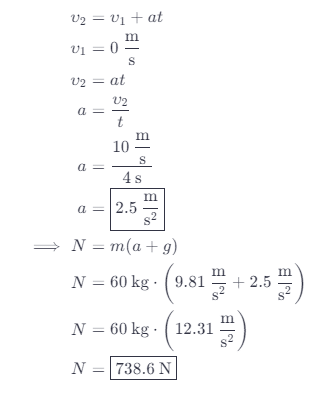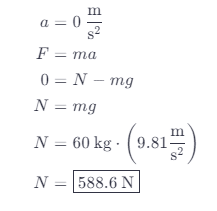# What Is The Passenger’s Weight While The Elevator Is Speeding Up?

We thoroughly check each answer to a question to provide you with the most correct answers. Found a mistake? Let us know about it through the REPORT button at the bottom of the page.

It takes the elevator in a skyscraper 4.0 s to reach its cruising speed of 10 m/s. A 60 kg passenger gets aboard on the ground floor. What is the passenger’s apparent weight

A. Before the elevator starts moving?

B. While the elevator is speeding up?

C. After the elevator reaches its cruising speed?

Contents

A) N = 588.6 N

B) N = 738.6 N

C) N = 588.6 N

## Explanation

Given values:

m = 60kg

t = 4s

V2 = 10m/s

The elevator is at rest. The only two forces acting are gravitational and the force of the normal reaction of the substrate.B) We will find the acceleration of the elevator from the equation:C) When the elevator reaches maximum speed, it no longer accelerates.